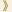HomeShaw Laureates2010Mathematical SciencesEssay

Mathematical analysis is concerned with the study of infinite processes, and the differential calculus of Newton and Leibniz lies at its heart.  It provided the foundation and the language for Newtonian mechanics and the whole of mathematical physics.  Over the past three centuries it has permeated much of mathematics and science.

Associated with the limiting process there are many technically difficult “estimates” or inequalities, of a combinatorial or algebraic nature, which prepare the ground and justify passing to the limit.  Such estimates are often extremely hard since they address some subtle and important aspect of the problem at hand.  Establishing this becomes a key step, opening the door to a wide variety of applications.

Over the past thirty years this study has undergone a mini-revolution in which a succession of hard problems of this nature have been solved, using a variety of novel techniques and ideas which often cross disciplinary boundaries and stimulate cross-fertilization.

Jean Bourgain is one of the leading analysts in the world today and he has played a major role in this revolution. He is much admired especially by those who make regular use of the multitude of powerful techniques that he has provided.  He has written over 350 papers, each of which is first rate, and a number of which contain solutions of central long-standing problems.

The fields in which he has made such fundamental contributions include harmonic analysis, functional analysis, ergodic theory, partial differential equations, mathematical physics, combinatorics and theoretical computer science.  Some of the well-known problems that he has solved include the embedding, with least distortion, of finite metric spaces in Hilbert space; extending the validity of Birkhoff’s ergodic theorem to very general sparse arithmetic sequences; and the boundedness in Lp of the circular maximum function in two dimensions.

He has also made a fundamental breakthrough in the study of the non-linear Schrödinger equation for the critical exponent defocusing case, introducing new tools which have led to significant progress on this difficult problem.

A whole area where Bourgain has led the way, and which deserves special mention, is the field of arithmetic combinatorics and its applications. A notable example is his solution of the “local” version of the Erdös-Volkmann conjecture.  The original conjecture asserts that any measurable subring of the real line has dimension either 0 or 1.  This was proved by Edgar and Miller in 2003 and, around the same time, Bourgain established the local version. This provides a sharp and powerful quantification of this phenomenon and is technically a tour de force.

In 2004 Bourgain, Katz and Tao proved their celebrated finite field analogue, known as the “Sum Product theorem”.  This is an elementary and fundamental quantification of the fact that finite fields have no subrings, and it measures a basic disjunction between the operations of addition and multiplication in a finite field. Bourgain has developed and extended this phenomenon making it into a theory.  A first application is to estimating algebro-geometric character sums.  For this the standard tool has been the famous solution of the Weil conjectures, established by Deligne using Grothendieck’s cohomology theory.  However for these methods to give non-trivial information one needs the Betti numbers of the corresponding varieties to be small compared to the size of the finite field.  What is remarkable about Bourgain’s results is that they give results even when the Betti numbers are big.

Another application of Bourgain’s theory, developed in collaboration with Gamburd is a proof of the expander conjecture of Lubotsky for the group SL2(Fp) and the spectral gap conjecture for elements in the group SU(2).  These are concerned with the spectra of the images, in high dimensional representations of these groups, of elements of their group rings.  They yield exponentially sharp equidistribution rates for random walks in these groups and are central to problems such as classical sieving in number theory and to aperiodic tilings of 3-dimensional space.

Bourgain has also developed some striking applications of his theory to theoretical computer science by giving a much sought after explicit construction of pseudorandom objects called extractors.  These, as well as the expanders, are basic building blocks used in fast derandomization algorithms.

Bourgain’s spectacular contributions to modern mathematics make him a very deserving winner of the 2010 Shaw Prize in the Mathematical Sciences.

Mathematical Sciences Selection Committee
The Shaw Prize

28 September 2010, Hong Kong